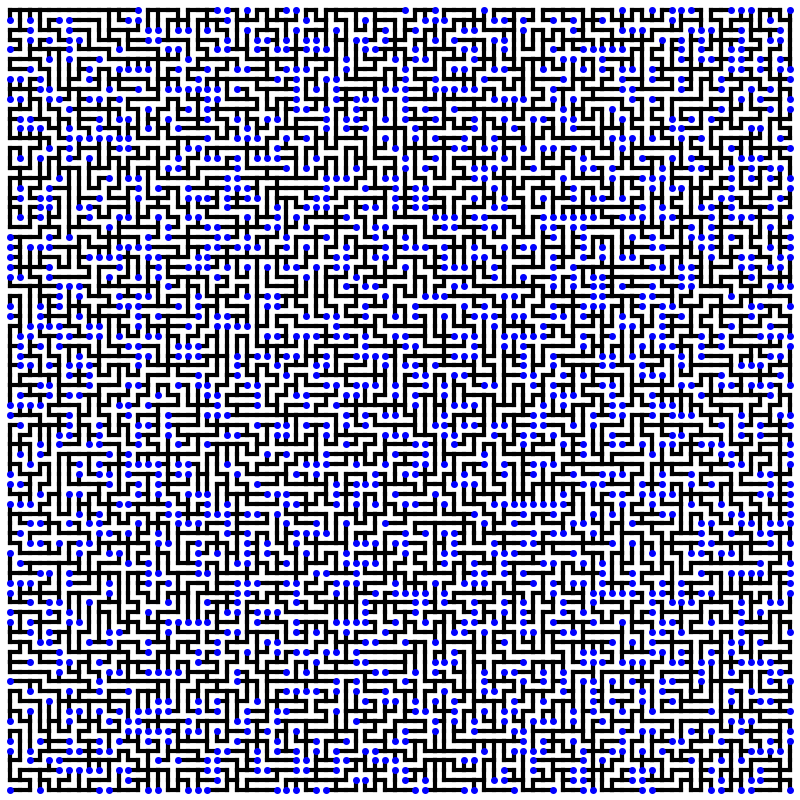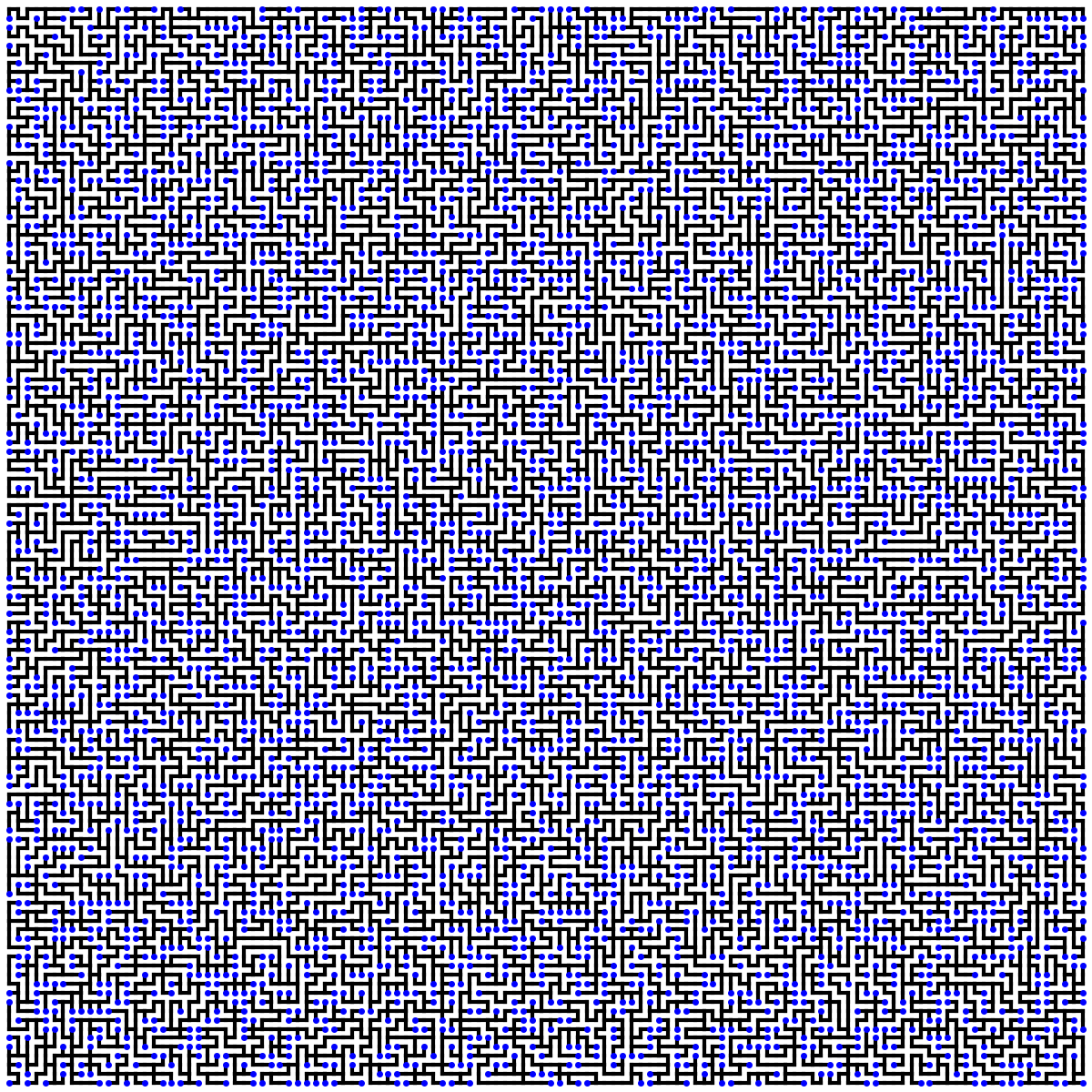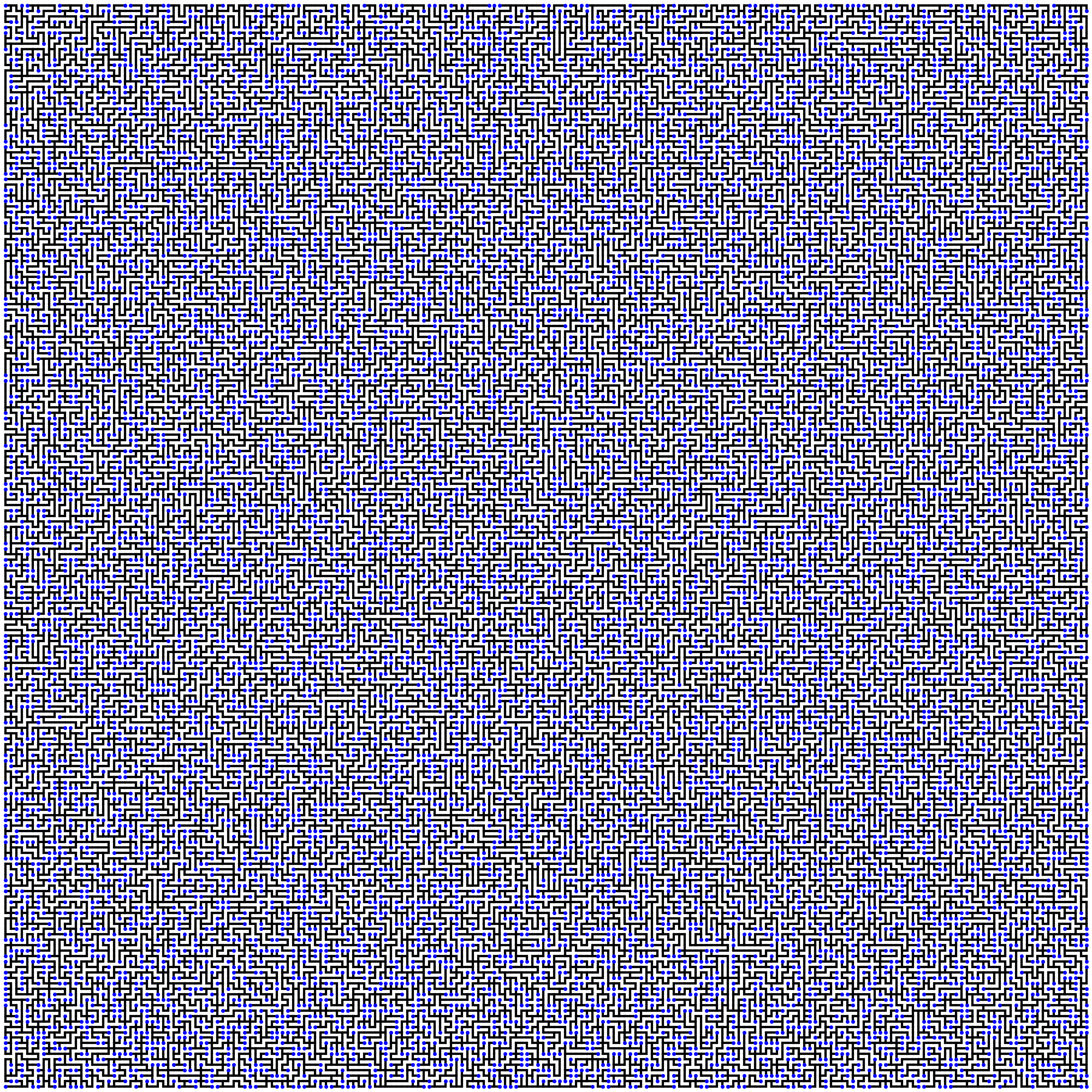# 二维随机游动 (二)：一个随机的完美迷宫分别有多少个死角、拐角、岔路和十字路口？

80x80, 0.294375 120x120, 0.294028 200x200, 0.29441：死角 $\dfrac{8}{\pi^2}(1-\dfrac{2}{\pi})$ 0.294
2：拐角 $\dfrac{4}{\pi}(2-\dfrac{9}{\pi}+\dfrac{12}{\pi^2})$ 0.447
3：岔路 $2(1-\dfrac{2}{\pi})(1-\dfrac{6}{\pi}+\dfrac{12}{\pi^2})$ 0.222
4：十字路口 $(\dfrac{4}{\pi}-1)(1-\dfrac{2}{\pi})^2$ 0.036

• $T$$G_n$ 的一个随机生成树 (服从均匀分布)，则任一边 $e$ 属于 $T$ 的概率 $\mathbb{P}(e\in T)=Y(e, e)$。特别当 $n\to\infty$$G_n\to\mathbb{Z}^2$$Y(e,e)\to\frac{1}{2}$。所以如果把 $\mathbb{Z}^2$ 看作一个电网络并在一条边 $e$ 的一端输入单位电流，将 $e$ 的另一端接地的话，那么恰好会有一半的电流直接从边 $e$ 流出，另外一半经过网络的其它部分后从 $e$ 的另一端流出。

• 仍然设 $T$ 是一个随机生成树，对任何 $k$ 条边 $e_1,\ldots,e_k$，定义 $k\times k$ 矩阵 $Y^{(k)}$$Y^{(k)}(i,j)=Y(e_i,e_j)$，则我们有如下结论 (转移电流矩阵定理)： $\mathbb{P}(e_1,\ldots,e_k \in T)=\det Y^{(k)}.$ 举个例子，在无穷大网格图 $\mathbb{Z}^2$ 中，考虑原点 $(0, 0)$ 相邻的四条边 $e_1=(1,0)$$e_2=(0, 1)$$e_3=(-1,0)$$e_4=(0, -1)$，则这四条边对应的转移电流矩阵为 $Y^{(4)}= \begin{pmatrix} \frac{1}{2} & \frac{1}{2}-\frac{1}{\pi} & \frac{2}{\pi}-\frac{1}{2} & \frac{1}{2}-\frac{1}{\pi}\\ \frac{1}{2}-\frac{1}{\pi} & \frac{1}{2} & \frac{1}{2}-\frac{1}{\pi} & \frac{2}{\pi}-\frac{1}{2}\\ \frac{2}{\pi}-\frac{1}{2} & \frac{1}{2}-\frac{1}{\pi} & \frac{1}{2} & \frac{1}{2}-\frac{1}{\pi}\\ \frac{1}{2}-\frac{1}{\pi} & \frac{2}{\pi}-\frac{1}{2} & \frac{1}{2}-\frac{1}{\pi} & \frac{1}{2} \end{pmatrix}.$ 于是原点的度数等于 4 的概率是 $\mathbb{P}(e_1,e_2,e_3,e_4\in\mathbb{T})=\det Y^{(4)}=(\frac{4}{\pi}-1)(1-\frac{2}{\pi})^2\approx 0.361.$ 如果是计算概率 $\mathbb{P}(e_1,e_2,e_3\notin T, e_4\in T)$ 呢？这有一个简单的办法，把 $e_1,e_2,e_3$ 对应的 $Y^{(4)}$ 的前三行全部取负值，给前三个对角元加上 1，保持第 4 行不变，得到 $Y^{(4)}_{\overline{123}4}= \begin{pmatrix} \frac{1}{2} & \frac{1}{\pi}-\frac{1}{2} & -\frac{2}{\pi}+\frac{1}{2} & \frac{1}{\pi}-\frac{1}{2}\\ \frac{1}{\pi}-\frac{1}{2} & \frac{1}{2} & \frac{1}{\pi}-\frac{1}{2} & -\frac{2}{\pi}+\frac{1}{2}\\ -\frac{2}{\pi}+\frac{1}{2} & \frac{1}{\pi}-\frac{1}{2} & \frac{1}{2} & \frac{1}{\pi}-\frac{1}{2}\\ \frac{1}{2}-\frac{1}{\pi} & \frac{2}{\pi}-\frac{1}{2} & \frac{1}{2}-\frac{1}{\pi} & \frac{1}{2}\end{pmatrix}.$$\mathbb{P}(e_1,e_2,e_3\notin T, e_4\in T)=\det Y^{(4)}_{\overline{123}4}=\frac{2}{\pi^2}-\frac{4}{\pi^3}.$ 于是原点是叶节点的概率是 $4\times\det Y^{(4)}_{\overline{123}4}\approx 0.2945$，这正是之前看到的。

计算其它容斥概率的步骤也都是类似的，只要在转移概率矩阵中将需要排除的边对应的行取负，然后在该行的对角元上加 1 即可。由 $\mathbb{Z}^2$ 对称性，其所有顶点的度数分布均相同。总之只要知道了转移电流矩阵 $Y$，我们就可以很容易得到任一顶点在 $T$ 中度数的分布，多方便！😄

注意虽然求解矩阵 $Y$ 需要 $\mathbb{Z}^2$ 的全局信息，但是计算顶点度数的分布却只需要 $Y$ 的局部信息。

# 1 图上的线性代数

$x,y\in V$ 是两个顶点，我们用 $x\sim y$ 表示 $x,y$ 是相邻的。由于本文主要考察边上的流函数，而流函数是有方向的，所以我们约定边集 $E$ 同时包含有序对 $(x,y)$$(y,x)$，即一条边作为有序对在 $E$ 中出现两次。当 $e=(x,y)$ 时，我们记 $-e=(y,x)$$e$反向边。并用记号 $E_{1/2}$ 表示对每个 $e$，在 $e$$-e$ 中任选一个组成的集合。此外我们称 $e^-=x$$e$尾部$e^+=y$$e$头部。注意在反向边 $-e$$x$ 变为头部，$y$ 变为尾部。

1. 定义梯度算子 $\nabla: \ell^2(V)\to\ell^{2}_{-}(E)$$(\nabla f)(x,y) = c(x, y)\big[f(x)-f(y)\big]=c(e)\big[f(e^-)-f(e^+)\big].$

2. 定义散度算子 $\mathrm{div}:\ell^{2}_{-}(E)\to\ell^2(V)$$(\mathrm{div}\,\theta)(x) = \frac{1}{c(x)}\sum_{y\sim x}\theta(x, y)=\frac{1}{c(x)}\sum_{e^-=x}\theta(e).$

3. 定义拉普拉斯算子 $\Delta:\ell^2(V)\to\ell^{2}(V)$$(\Delta f)(x) = (\mathrm{div}\,\nabla f)(x) = f(x)-\sum_{y\sim x}\frac{c(x,y)}{c(x)}f(y).$ 如果 $(\Delta f)(x)=0$$x\in V$ 成立，我们就称 $f$$x$ 处是调和的。

$\theta\in\lozenge$，定义 $\theta$ 的支集为 $\mathrm{supp}(\theta)=\{e\in E\,|\,\theta(e)\ne0\}.$ 注意如果 $\mathrm{supp}(\theta)$ 非空的话，则它必然包含一个回路，否则 $\mathrm{supp}(\theta)$ 构成的子图中必然有一个叶顶点 (度数恰好为 1)，从而这一点处的散度不可能为 0，矛盾！于是 $\mathrm{supp}(\theta)$ 包含一个回路 $C$，我们可以选择合适的常数 $\alpha$ 使得 $\widetilde{\theta}=\theta-\alpha f^C$ 在某个 $e\in C$ 上为 0，则 $\widetilde{\theta}$ 的支集严格小于 $\theta$。对 $\widetilde{\theta}$ 重复此操作一直下去必然可以在有限次后将支集变为空集，这就证明了 $\theta$ 可以表示为形如 $f^C$ 的线性组合。

# 2 网络中的流

1. $(\mathrm{div}\,\theta)(a)\geq 0$
2. 对任何 $x\notin \{a\}\cup Z$$(\mathrm{div}\,\theta)(x)=0$

$a$ 叫做 $\theta$ 的源点，$Z$ 叫做 $\theta$ 的汇点。

1. 你可以把 $a$ 理解为电源正极，$Z$ 理解为网络接地的部分。
2. 流函数要求对任何 $x\notin \{a\}\cup Z$$(\mathrm{div}\,\theta)(x)=0$ 等价于物理中的基尔霍夫定律：任何中间节点的流量守恒。
3. 电流函数要求 $\theta=\nabla g\in\bigstar$ 等价于物理中的欧姆定律，即电流 $\theta$ 等于电势差 $\nabla g$ 除以电阻 $r(e)$

# 3 电网络物理量的概率解释

• 我们考虑从一点 $x$ 出发，转移概率为 $P(x,y)=c(x,y)/c(x)$ 的随机游动 $\{X_t,t\geq0\}$，其中 $X_0=x$。这个随机游动是可逆的 Markov 链，以 $\{c(x),x\in V\}$ 为平稳测度：$c(x)P(x,y)=c(y)P(y,x)$

• 我们同时考虑在 $a$ 点接上电源正极，并将 $Z$ 接地，则电流从 $a$ 流入并从 $Z$ 流出。我们将建立随机游动和这一电网络之间的联系。

## 电流的概率解释

$\mathbb{P}(a\to Z)=\mathbb{P}_a(\tau_Z < \tau_a^+)$ 为从 $a$ 出发的随机游动在返回 $a$ 之前先到达 $Z$ 的概率。此概率称作从 $a$$Z$逃逸概率

1. $v(x) = \mathcal{G}_Z(a, x)/ c(x)$
2. $i(x,y)=\mathbb{E}_a\left[N_{x\to y}^Z - N_{y\to x}^Z\right]$

1. 首先边界条件给出了 $\mathcal{G}_Z(a, Z)=0$。此外 $\mathcal{G}_Z(a, a)$ 是一个每次试验成功概率为 $\mathbb{P}(a\to Z)$ 的几何分布的随机变量的期望，故 $\mathcal{G}_Z(a, a)=\frac{1}{\mathbb{P}(a\to Z)},$ 再由上面的引理，有 $\frac{\mathcal{G}_Z(a, a)}{c(a)}=\frac{1}{c(a)\mathbb{P}(a\to Z)}=v(a).$ 所以 $\mathcal{G}_Z(a, x)/c(x)$ 在边界上与电势函数是一致的，只要再证明在任意中间节点 $x$ 的调和性质。注意随机游动必然是从与 $x$ 相邻的顶点走过去访问 $x$，所以 $\frac{\mathcal{G}_Z(a, x)}{c(x)}=\frac{1}{c(x)}\sum_{y\sim x}\mathbb{E}_a[N_{y\to x}^Z].$ 另一方面 \begin{align*} \mathbb{E}_a[N_{y\to x}^Z]&= \mathbb{E}_a\left[\sum_{t=0}^{\tau_Z}1_{\{X_t=y,X_{t+1}=x\}}\right]\\&= \sum_{t=0}^{\tau_Z}\mathbb{P}\left[X_t=y,X_{t+1}=x\right]\\&= \sum_{t=0}^{\tau_Z}\mathbb{P}(X_t=y)P(y,x)\\&= P(y,x)\mathbb{E}_a\left[\sum_{t=0}^{\tau_Z}1_{\{X_t=y\}}\right]\\&= P(y,x)\mathcal{G}_Z(a,y). \end{align*} \label{eq:cross} 所以代入求和后有 $\frac{1}{c(x)}\sum_{y\sim x}\mathbb{E}_a[N_{y\to x}^Z]=\frac{1}{c(x)}\sum_{y\sim x}P(y,x)\mathcal{G}_Z(a,y)=\sum_{y\sim x}P(x,y)\frac{\mathcal{G}_Z(a,y)}{c(y)}.$ 其中我们使用了 Markov 链的可逆性：$c(x)P(x,y)=c(y)P(y,x)$。这就证明了 $\mathcal{G}_Z(a, x)/c(x)$ 在中间节点的调和性，从而它就是电势函数，这证明了 1。

2. 直接验证即可： \begin{align*}\mathbb{E}_a\left[N_{x\to y}^Z - N_{y\to x}^Z\right]&=P(x,y)\mathcal{G}_Z(a,x)-P(y,x)\mathcal{G}_Z(a,y)\\&=P(x,y)c(x)v(x) - P(y,x)c(y)v(y)\\&=c(x,y)(v(x)-v(y))\\&=i(x,y).\end{align*}

# 4 Wilson 均匀生成树算法

Wilson 算法的步骤如下：

1. 任取一个根节点 $r$，维护一个树 $T$，初始时 $T=\{r\}$
2. 任取一个顶点 $v\notin T$，从 $v$ 出发作 $G$ 上的擦圈随机游动，直到随机游动撞到 $T$ 为止，然后将随机游动的路径 $p$ 加入 $T$ 中：$T=T\cup p$
3. 重复步骤 2 直到 $T$ 是一个生成树为止。

Wilson 算法正确性的证明比较繁琐，这里不再赘述，你可以参考我之前的一篇文章。初次遇到这个算法的话可以先跳过证明，只记住结论。这个算法的重点就是初始时的根节点是可以任意选取的，每次进行擦圈的随机游动时选择的出发顶点也可以是任意的，这都不影响得到的生成树 $T$ 的分布。

1. 是从边 $(x,y)$ 走到 $y$ 的，此随机游动的路径属于 $T$，自然也有 $e\in T$
2. 是从其它边走到 $y$ 的，则此随机游动的路径属于 $T$ 但不可能有 $e\in T$，否则 $T$ 中会出现回路。

# 5 图的收缩

$\mathcal{N}=(G,c)$ 是一个网络，$F\subset E$ 是边的子集且 $F$ 不含回路 (不考虑边的方向)，$G$ 关于 $F$ 的图收缩是一个图变换，它把每个 $F$ 中的每条边 $f$ 的两个端点合并为一个新顶点，并将 $f$ 变成新顶点的一条自边 (loop)。这样得到的新图记作 $G/F$。注意当一条边 $e\notin F$ 如果和 $F$ 中的边构成回路的话，它在 $G/F$ 中也会变成自边。1. $I^e\in \bigstar$。(Ohm 定律)
2. $I^e\downharpoonright F=0$。(任何 $F$ 中的边两端等势)
3. 流量限制：
• $F$ 的任何连通成分 $F_C$，若 $F_C$ 不包含 $e$ 的任何端点，则从 $F_C$ 净流出的电流量为 0。
• $F$ 的任何连通成分 $F_C$，若 $e^-\in F_C (e^+\in F_C)$ 则从 $F_C$ 净流出的电流为 1 (-1)。
• $I^e$$G$ 的其它顶点 $x\notin e\cup F$ 处流量守恒。

Fact：若 $U,V$ 是内积空间的子空间且 $U\supseteq V^\bot$，则 $U = V^\bot\oplus (U\cap V) = V^\bot\oplus P_VU.$ 其中 $P_V$ 是从全空间到 $V$ 的正交投影算子。

$\chi^F=\{\chi^f,f\in F\}$ 为所有 $f\in F$ 对应的单位流 $\chi^F$ 张成的子空间，$Z=\{i^f,f\in F\}=P_\bigstar\chi^F$，则 $Z\subset\bigstar$。对 $U=\bigstar$$V=Z^\bot$ 使用上述事实得到 $\bigstar = Z \oplus P_{Z}^\bot\bigstar.$推论 2.2 可知 $I^e\downharpoonright F=0$ 等价于 $(I^e,i^f)_E=0$ 对任何 $f\in F$ 成立，即 $I^e\in Z^\bot$，而 $I^e\in\bigstar$，从而 $I^e\in \bigstar\cap Z^{\bot}=P_Z^\bot\bigstar$，因此这个 $I^e$ 是什么就很清楚了，它正是电流函数 $i^e$$Z^\bot$ 上的正交投影分量： $I^e = P_Z^\bot i^e.$ 稍微麻烦一点的是验证 $I^e$ 满足条件 3。记 $I^e = i^e - \sum_{f\in F}\alpha_f f.$ 则在情形 1 中，每个 $i^f$$f$ 两端的净流出量抵消，$F_C$ 总的净流出量为 0；在情形 2 中边 $e$ 只有一端在 $F$ 中，其贡献的净流出量为 $+1$ 或者 $-1$，其它边 $i^f$$f$ 两端的净流出量抵消，$F_C$ 总的净流出量为 $\pm1$；在情形 3 中 $i^e$ 和所有 $i^f$ 在顶点处的净流出量都是 0，$F_C$ 总的净流出量为 0。

# 6 转移电流定理

$M^{(r)},M^{(r-1)},P$ 在所有 $i\ne r$ 的行都相等，但是 $M^{(r)}$$M^{(r-1)}$ 的第 $r$ 行之和等于 $P$$r$ 行，于是根据行列式的多重线性性质， $\det M^{(r)}+\det M^{(r-1)}=\det P.$$\det P$ 按照 $P$ 的第 $r$ 行展开并对 $\{e_1,\ldots,e_{r-1},e_{r+1},\ldots,e_k\}$ 利用归纳假设，得到 $\det P=\mathbb{P}(e_1,\ldots,e_{r-1}\notin T, e_{r+1},\ldots,e_k\in T).$ 又对 $\{e_1,\ldots,e_{r-1},e_r,\ldots,e_k\}$ 利用归纳假设得到 $\det M^{(r-1)} = \mathbb{P}(e_1,\ldots,e_{r-1}\notin T, e_{r},\ldots,e_k\in T)$ 相减即得结论。

1. 如果 $\{e_1,\ldots,e_k\}$ 中包含某个回路 $C$ 的话，我们可以适当重排它们并选取 $a_i\in\{+1,-1,0\}$ 使得 $\sum_i a_i\chi^{e_i}=f_C\in\lozenge$，这里对不属于 $C$$e_i$$a_i=0$，对属于 $C$$e_i$ 通过选择 $a_i=\pm1$ 使得它们的方向为依次首尾相接。则根据推论 2.2 $Y^{(k)}$ 的行是线性相关的，从而 $\mathbb{P}(e_1,\ldots,e_k\in T)=\det Y^{(k)}=0$，结论成立。

2. 如果 $\{e_1,\ldots,e_k\}$ 中不包含回路的话，对 $k$ 归纳，定理 4.1 已经给出了 $k=1$ 的情形，所以可以假设 $k>1$ 且结论对所有小于 $k$ 的情形成立。我们注意到 $Y^{(k)}$ “差不多”是一个 Gram 矩阵 (见之前的推论)： $Y^{(k)}(i,j)=Y(e_i,e_j)=c(e_j)(i^{e_i},i^{e_j})_E.$$Y^{(k)}$ 不过是对每个 $j$，在 Gram 矩阵 $I^{(k)}=\big((i^{e_i},i^{e_j})\big)_{1\leq i,j\leq k}$ 的第 $j$ 列上乘以 $c(e_j)$ 得到的，所以 $\det Y^{(k)} = \left(\prod_{i=1}^k c(e_i)\right)\det I^{(k)}.$ 回忆内积空间中 Gram 矩阵的行列式的几何意义是其张成的平行多面体的体积的平方，它等于 $\{i^{e_1},\ldots,i^{e_{k-1}}\}$ 张成的底面 $Z$ 面积平方乘以 $i^{e_k}$ 到这个底面距离的平方： $\det I^{(k)} = \big\|P_Z^\bot i^{e_k}\big\|^2\det I^{(k-1)}.$ 其中 $Z=\mathrm{span}\{i^{e_1},\ldots,i^{e_{k-1}}\}$$P_Z^\bot$ 是到 $Z$ 的正交补空间 $Z^\bot$ 的投影算子。把 $\prod_{i=1}^kc(e_i)$ 乘回去就得到 $\det Y^{(k)} = c(e_k) \big\|P_Z^\bot i^{e_k}\big\|^2 \det Y^{(k-1)}.$ 另一方面根据条件概率公式有 $\mathbb{P}(e_1,\ldots,e_k\in T)= \mathbb{P}(e_k\in T\,\big|\, e_1,\ldots,e_{k-1}\in T)\cdot \mathbb{P}(e_1,\ldots,e_{k-1}\in T).$ 而根据定理 5.1 的结论 $\mathbb{P}(e_k\in T\,\big|\, e_1,\ldots,e_{k-1}\in T) = c(e_k) \big\|P_Z^\bot i^{e_k}\big\|^2,$ 从而 $\det Y^{(k)}$$\mathbb{P}(e_1,\ldots,e_k\in T)$ 有相同的初始条件和递推关系，从而它们相等，转移电流定理得证。

# 7 回答本文开头的问题1. Lyons, Russell, and Peres, Yuval. 2016. Probability on Trees and Networks. Cambridge Series in Statistical and Probabilistic Mathematics, vol. 42.↩︎

2. Richard Stanley. 2013. Algebraic Combinatorics: Walks, Trees, Tableaux, and More. Undergraduate Texts in Mathematics. Springer-Verlag New York.↩︎